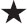# User ForumSubject :IMO    Class : Class 5is twice the difference between the 6th and the 10th multiple of 7.
Find.

A 38
B 56
C 60
D 28

this is 56

Class : Class 1

(B): 6th multiple of 7 = 42
10th multiple of 7 = 70
Now,= 2 × (70 - 42) = 2 × 28 = 56

## Ans 2:

Class : Class 1
Ans 1 I think u have copied this from the solution. This is the same thing in the solution. No offence.

## Ans 3:

Class : Class 1
And I did not understand the solution. So, can u explain it better?

## Ans 4:

Class : Class 7
6th multiple of 7 = 4210th multiple of 7 = 7042 - 70 = 2828* 2 = 56So, the correct answer is B.